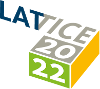#The 39th International Symposium on Lattice Field Theory (Lattice 2022)

Aug 8 – 13, 2022
Hörsaalzentrum Poppelsdorf
Europe/Berlin timezone

## Symmetry breaking in an extended-O(2) model

Aug 10, 2022, 3:40 PM
20m
CP1-HSZ/1.004 (CP1-HSZ) - HS7 (CP1-HSZ)

### CP1-HSZ/1.004 (CP1-HSZ) - HS7

#### CP1-HSZ

70
Show room on map
Oral Presentation Algorithms (including Machine Learning, Quantum Computing, Tensor Networks)

### Speaker

Leon Hostetler (Michigan State University)

### Description

Motivated by attempts to quantum simulate lattice models with continuous Abelian symmetries using discrete approximations, we consider an extended-O(2) model that differs from the ordinary O(2) model by an explicit symmetry breaking term. Its coupling allows to smoothly interpolate between the O(2) model (zero coupling) and a $q$-state clock model (infinite coupling). In the latter case, a $q$-state clock model can also be defined for non-integer values of $q$. Thus, such a limit can also be considered as an analytic continuation of an ordinary $q$-state clock model to non-integer $q$. The phase diagram of the extended-O(2) model in the infinite coupling limit was established in our previous work, where it was shown that for non-integer $q$, there is a second-order phase transition at low temperature and a crossover at high temperature. In this work, we investigate the model at finite values of the coupling using Monte Carlo and tensor methods. The results may be relevant for configurable Rydberg-atom arrays.

### Primary authors

Leon Hostetler (Michigan State University) Ryo Sakai (Syracuse University) Jin Zhang (The University of Iowa) Judah Unmuth-Yockey (Fermilab) Alexei Bazavov (Michigan State University) Yannick Meurice (University of Iowa)